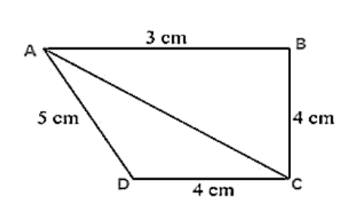# Find the area of the quadrilateral ABCD in which AB = 3 cm,Question:

Find the area of the quadrilateral ABCD in which AB = 3 cm, BC = 4 cm, CD = 4 cm, DA = 5 cm and AC = 5 cm.

Solution:

For triangle ABC

$A C^{2}=B C^{2}+A B^{2}$

25 = 9 + 16

So, triangle ABC is a right angle triangle right angled at point R

Area of triangle ABC = 12 × AB × BC

= 1/2 × 3 × 4

$=6 \mathrm{~cm}^{2}$

Perimeter = 2s = AC + CD + DA

2s = 5 cm+ 4 cm+ 5 cm

2s = 14 cm

s = 7 cm

By using Heron's Formula

Area of the triangle $\mathrm{CAD}=\sqrt{\mathrm{s} \times(\mathrm{s}-\mathrm{a}) \times(\mathrm{s}-\mathrm{b}) \times(\mathrm{s}-\mathrm{c})}$

$=\sqrt{7 \times(7-5) \times(7-4) \times(7-5)}$

$=9.16 \mathrm{~cm}^{2}$

Area of ABCD = Area of ABC + Area of CAD

$=(6+9.16) \mathrm{cm}^{2}$

$=15.16 \mathrm{~cm}^{2}$